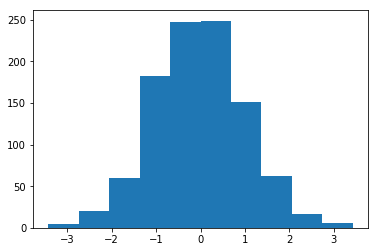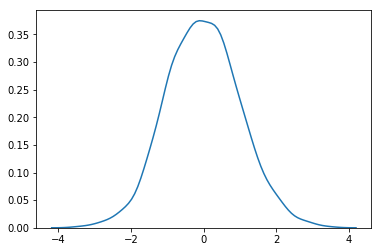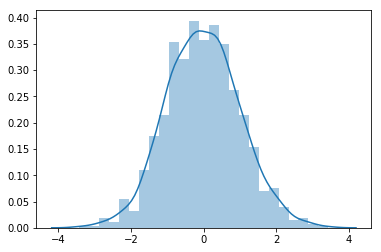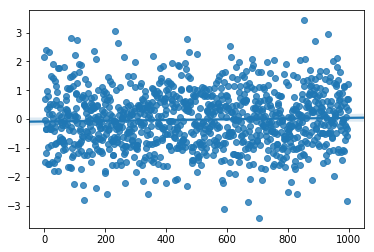In :
import numpy as np
import matplotlib.pyplot as plt
import seaborn as sns
x = np.random.randn(1000)  # sample from "standard normal" distribution.

In :
plt.hist(x)
plt.show()In :
sns.kdeplot(x)  # kernel density estimation
plt.show()In :
# Flexibly plot a univariate distribution of observations.
# looks similar with hist + kde
sns.distplot(x)
plt.show()In :
# Plot data and a linear regression model fit.
sns.regplot(np.arange(0, x.size), x)
plt.show()